# THYRISTOR D.C. DRIVES – GENERAL (Motors And Drives)

For motors up to a few kilowatts the armature converter can be supplied from either single-phase or three-phase mains, but for larger motors three-phase is always used. A separate thyristor or diode rectifier is used to supply the field of the motor: the power is much less than the armature power, so the supply is often single-phase, as shown in Figure 4.1.
The arrangement shown in Figure 4.1 is typical of the majority of d.c. drives and provides for closed-loop speed control. The function of the two control loops will be explored later, but readers who are not familiar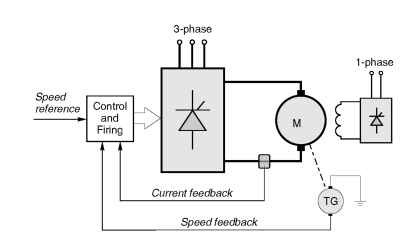Figure 4.1 Schematic diagram of speed-controlled d.c. motor drive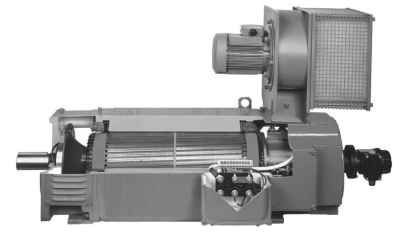Plate 4.1 High performance force-ventilated d.c. motor. The motor is of all-laminated construction and designed for use with a thyristor converter. The small blower motor is an induction machine that runs continuously, thereby allowing the main motor to maintain full torque at low speed without overheating. (Photo courtesy of Control Techniques)
with the basics of feedback and closed-loop systems may find it helpful to read through the Appendix at this point.
The main power circuit consists of a six-thyristor bridge circuit (as discussed in topic 2), which rectifies the incoming a.c. supply to produce a d.c. supply to the motor armature. The assembly of thyristors, mounted on a heatsink, is usually referred to as the ‘stack’. By altering the firing angle of the thyristors the mean value of the rectified voltage can be varied, thereby allowing the motor speed to be controlled.
We saw in topic 2 that the controlled rectifier produces a crude form of d.c. with a pronounced ripple in the output voltage. This ripple component gives rise to pulsating currents and fluxes in the motor, and in order to avoid excessive eddy-current losses and commutation problems, the poles and frame should be of laminated construction. It is accepted practice for motors supplied for use with thyristor drives to have laminated construction, but older motors often have solid poles and/or frames, and these will not always work satisfactorily with a rectifier supply. It is also the norm for drive motors to be supplied with an attached ‘blower’ motor as standard. This provides continuous through ventilation and allows the motor to operate continuously at full torque even down to the lowest speeds without overheating.
Low power control circuits are used to monitor the principal variables of interest (usually motor current and speed), and to generate appropriate firing pulses so that the motor maintains constant speed despite
variations in the load. The ‘speed reference’ (Figure 4.1) is typically an analogue voltage varying from 0 to 10 V, and obtained from a manual speed-setting potentiometer or from elsewhere in the plant.
The combination of power, control, and protective circuits constitutes the converter. Standard modular converters are available as off-the-shelf items in sizes from 0.5 kW up to several hundred kW, while larger drives will be tailored to individual requirements. Individual converters may be mounted in enclosures with isolators, fuses etc., or groups of converters may be mounted together to form a multi-motor drive.

### Motor operation with converter supply

The basic operation of the rectifying bridge has been discussed in topic 2, and we now turn to the matter of how the d.c. motor behaves when supplied with ‘d.c.’ from a controlled rectifier.
By no stretch of imagination could the waveforms of armature voltage looked at in topic 2 (e.g. Figure 2.12) be thought of as good d.c., and it would not be unreasonable to question the wisdom of feeding such an unpleasant looking waveform to a d.c. motor. In fact it turns out that the motor works almost as well as it would if fed with pure d.c., for two main reasons. Firstly, the armature inductance of the motor causes the waveform of armature current to be much smoother than the waveform of armature voltage, which in turn means that the torque ripple is much less than might have been feared. And secondly, the inertia of the armature is sufficiently large for the speed to remain almost steady despite the torque ripple. It is indeed fortunate that such a simple arrangement works so well, because any attempt to smooth-out the voltage waveform (perhaps by adding smoothing capacitors) would prove to be prohibitively expensive in the power ranges of interest.

### Motor current waveforms

For the sake of simplicity we will look at operation from a single-phase (2-pulse) converter, but similar conclusions apply to the 6-pulse converter. The voltage (Va) applied to the motor armature is typically as shown in Figure 4.2(a): as we saw in topic 2, it consists of rectified ‘chunks’ of the incoming mains voltage, the precise shape and average value depending on the firing angle.
The voltage waveform can be considered to consist of a mean d.c. level (Vdc), and a superimposed pulsating or ripple component which we can denote loosely as Vac. The mean voltage Vdc can be altered by varying the firing angle, which also incidentally alters the ripple (i.e. Vac).
The ripple voltage causes a ripple current to low in the armature, but because of the armature inductance, the amplitude of the ripple current is small. In other words, the armature presents a high impedance to a.c. voltages. This smoothing effect of the armature inductance is shown in Figure 4.2(b), from which it can be seen that the current ripple is relatively small in comparison with the corresponding voltage ripple. The average value of the ripple current is of course zero, so it has no effect on the average torque of the motor. There is nevertheless a variation in torque every half-cycle of the mains, but because it is of small amplitude and high frequency the variation in speed (and hence back e.m.f., E ) will not usually be noticeable.
The current at the end of each pulse is the same as at the beginning, so it follows that the average voltage across the armature inductance (L)is zero. We can therefore equate the average applied voltage to the sum of the back e.m.f. (assumed pure d.c. because we are ignoring speed fluctuations) and the average voltage across the armature resistance, to yield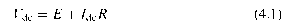which is exactly the same as for operation from a pure d.c. supply. This is very important, as it underlines the fact that we can control the mean motor voltage, and hence the speed, simply by varying the converter delay angle.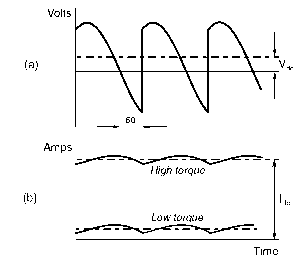Figure 4.2 Armature voltage (a) and armature current (b) waveforms for continuous-current operation of a d.c. motor supplied from a single-phase fully-controlled thyristor converter, with firing angle of 60°
The smoothing effect of the armature inductance is important in achieving successful motor operation: the armature acts as a low-pass filter, blocking most of the ripple, and leading to a more or less constant armature current. For the smoothing to be effective, the armature time-constant needs to be long compared with the pulse duration (half a cycle with a 2-pulse drive, but only one sixth of a cycle in a 6-pulse drive). This condition is met in all 6-pulse drives, and in many 2-pulse ones. Overall, the motor then behaves much as it would if it was supplied from an ideal d.c. source (though the I2R loss is higher than it would be if the current was perfectly smooth).
The no-load speed is determined by the applied voltage (which depends on the firing angle of the converter); there is a small drop in speed with load and as we have previously noted, the average current is determined by the load. In Figure 4.2, for example, the voltage waveform in (a) applies equally for the two load conditions represented in (b), where the upper current waveform corresponds to a high value of load torque while the lower is for a much lighter load; the speed being almost the same in both cases. (The small difference in speed is due to IR as explained in topic 3). We should note that the current ripple remains the same – only the average current changes with load. Broadly speaking, therefore, we can say that the speed is determined by the converter firing angle, which represents a very satisfactory state because we can control the firing angle by low-power control circuits and thereby regulate the speed of the drive.
The current waveforms in Figure 4.2(b) are referred to as ‘continuous’, because there is never any time during which the current is not lowing. This ‘continuous current’ condition is the norm in most drives, and it is highly desirable because it is only under continuous current conditions that the average voltage from the converter is determined solely by the firing angle, and is independent of the load current. We can see why this is so with the aid of Figure 2.7, imagining that the motor is connected to the output terminals and that it is drawing a continuous current. For half of a complete cycle, the current will low into the motor from T1 and return to the mains via T4, so the armature is effectively switched across the supply and the armature voltage is equal to the supply voltage, which is assumed to be ideal, i.e. it is independent of the current drawn. For the other half of the time, the motor current lows from T2 and returns to the supply via T3, so the motor is again hooked-up to the supply, but this time the connections are reversed. Hence the average armature voltage – and hence to a first approximation the speed – are defined once the firing angle is set.

### Discontinuous current

We can see from Figure 4.2 that as the load torque is reduced, there will come a point where the minima of the current ripple touches the zero-current line, i.e. the current reaches the boundary between continuous and discontinuous current. The load at which this occurs will also depend on the armature inductance, because the higher the inductance the smoother the current (i.e. the less the ripple). Discontinuous current mode is therefore most likely to be encountered in small machines with low inductance (particularly when fed from two-pulse converters) and under light-load or no-load conditions.
Typical armature voltage and current waveforms in the discontinuous mode are shown in Figure 4.3, the armature current consisting of discrete pulses of current that occur only while the armature is connected to the supply, with zero current for the period (represented by U in Figure 4.3) when none of the thyristors are conducting and the motor is coasting free from the supply.
The shape of the current waveform can be understood by noting that with resistance neglected, equation (3.7) can be rearranged as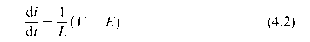which shows that the rate of change of current (i.e. the gradient of the lower graph in Figure 4.3) is determined by the instantaneous difference between the applied voltage V and the motional e.m.f. E. Values of (V — E) are shown by the vertical hatchings in Figure 4.3, from which it can be seen that if V > E, the current is increasing, while if V < E, the current is falling. The peak current is thus determined by the area of the upper or lower shaded areas of the upper graph.
The firing angle in Figures 4.2 and 4.3 is the same, at 60°, but the load is less in Figure 4.3 and hence the average current is lower (though, for the sake of the explanation offered below the current axis in Figure 4.3 is expanded as compared with that in Figure 4.2). It should be clear by comparing these figures that the armature voltage waveforms (solid lines) differ because, in Figure 4.3, the current falls to zero before the next firing pulse arrives and during the period shown as U the motor loats free, its terminal voltage during this time being simply the motional e.m.f. (E). To simplify Figure 4.3 it has been assumed that the armature resistance is small and that the corresponding volt-drop (IaRa) can be ignored. In this case, the average armature voltage (Vdc) must be equal to the motional e.m.f., because there can be no average voltage across the armature inductance when there is no nett change in the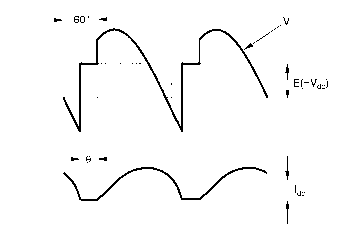Figure 4.3 Armature voltage current waveforms for discontinuous-current operation of a d.c. motor supplied from a single-phase fully-controlled thyristor converter, with firing angle of60°
current over one pulse: the hatched areas – representing the volt-seconds in the inductor – are therefore equal.
The most important difference between Figures 4.2 and 4.3 is that the average voltage is higher when the current is discontinuous, and hence the speed corresponding to the conditions in Figure 4.3 is higher than in 4.2 despite both having the same firing angle. And whereas in continuous mode a load increase can be met by an increased armature current without affecting the voltage (and hence speed), the situation is very different when the current is discontinuous. In the latter case, the only way that the average current can increase is when speed (and hence E) falls so that the shaded areas in Figure 4.3 become larger.
This means that from the user’s viewpoint the behaviour of the motor in discontinuous mode is much worse than in the continuous current mode, because as the load torque is increased, there is a serious drop in speed. The resulting torque-speed curve therefore has a very unwelcome ‘droopy’ characteristic in the discontinuous current region, as shown in Figure 4.4, and in addition the I2R loss is much higher than it would be with pure d.c.
Under very light or no-load conditions, the pulses of current become virtually non-existent, the shaded areas in Figure 4.3 become very small and the motor speed reaches a point at which the back e.m.f. is equal to the peak of the supply voltage.
It is easy to see that inherent torque-speed curves with sudden discontinuities of the form shown in Figure 4.4 are very undesirable. If for example the firing angle is set to zero and the motor is fully loaded, its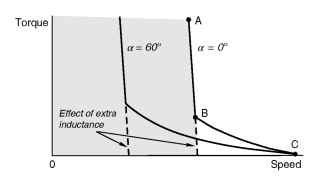Figure 4.4 Torque-speed curves illustrating the undesirable ‘droopy’ characteristic associated with discontinuous current. The improved characteristic (shown dotted) corresponds to operation with continuous current
speed will settle at point A, its average armature voltage and current having their full (rated) values. As the load is reduced, current remaining continuous, there is the expected slight rise in speed, until point B is reached. This is the point at which the current is about to enter the discontinuous phase. Any further reduction in the load torque then produces a wholly disproportionate – not to say frightening – increase in speed, especially if the load is reduced to zero when the speed reaches point C.
There are two ways by which we can improve these inherently poor characteristics. Firstly, we can add extra inductance in series with the armature to further smooth the current waveform and lessen the likelihood of discontinuous current. The effect of adding inductance is shown by the dotted lines in Figure 4.4. And secondly, we can switch from a single-phase converter to a 3-phase converter which produces smoother voltage and current waveforms, as discussed in topic 2.
When the converter and motor are incorporated in a closed-loop control the user should be unaware of any shortcomings in the inherent motor/converter characteristics because the control system automatically alters the firing angle to achieve the target speed at all loads. In relation to Figure 4.4, for example, as far as the user is concerned the control system will continue operation to the shaded region, and the fact that the motor is theoretically capable of running unloaded at the high speed corresponding to point C is only of academic interest.

### Converter output impedance: overlap

So far we have tacitly assumed that the output voltage from the converter was independent of the current drawn by the motor, and depended only
on the delay angle a. In other words we have treated the converter as an ideal voltage source.
In practice the a.c. supply has a finite impedance, and we must therefore expect a volt-drop which depends on the current being drawn by the motor. Perhaps surprisingly, the supply impedance (which is mainly due to inductive leakage reactances in transformers) manifests itself at the output stage of the converter as a supply resistance, so the supply volt-drop (or regulation) is directly proportional to the motor armature current.
It is not appropriate to go into more detail here, but we should note that the effect of the inductive reactance of the supply is to delay the transfer (or commutation) of the current between thyristors; a phenomenon known as overlap. The consequence of overlap is that instead of the output voltage making an abrupt jump at the start of each pulse, there is a short period when two thyristors are conducting simultaneously. During this interval the output voltage is the mean of the voltages of the incoming and outgoing voltages, as shown typically in Figure 4.5. It is important for users to be aware that overlap is to be expected, as otherwise they may be alarmed the irst time they connect an oscilloscope to the motor terminals. When the drive is connected to a ‘stiff’ (i.e. low impedance) industrial supply the overlap will only last for perhaps a few microseconds, so the ‘notch’ shown in Figure 4.5 would be barely visible on an oscilloscope. topics always exaggerate the width of the overlap for the sake of clarity, as in Figure 4.5: with a 50 or 60 Hz supply, if the overlap lasts for more than say 1 ms, the implication is that the supply system impedance is too high for the size of converter in question, or conversely, the converter is too big for the supply.
Returning to the practical consequences of supply impedance, we simply have to allow for the presence of an extra ‘source resistance’ in series with the output voltage of the converter. This source resistance is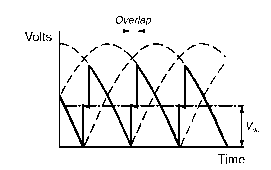Figure 4.5 Distortion of converter output voltage waveform caused by rectifier overlap in series with the motor armature resistance, and hence the motor torque-speed curves for each value of a have a somewhat steeper droop than they would if the supply impedance was zero.

So far we have looked at the converter as a rectifier, supplying power from the a.c. mains to a d.c. machine running in the positive direction and acting as a motor. As explained in topic 3, this is known as one-quadrant operation, by reference to quadrant 1 of the complete torque-speed plane shown in Figure 3.16.
But suppose we want to run the machine as a motor in the opposite direction, with negative speed and torque, i.e. in quadrant 3; how do we do it? And what about operating the machine as a generator, so that power is returned to the a.c. supply, the converter then ‘inverting’ power rather than rectifying, and the system operating in quadrant 2 or quadrant 4. We need to do this if we want to achieve regenerative braking. Is it possible, and if so how?
The good news is that as we saw in topic 3 the d.c. machine is inherently a bidirectional energy converter. If we apply a positive voltage V greater than E, a current flows into the armature and the machine runs as a motor. If we reduce V so that it is less than E, the current, torque and power automatically reverse direction, and the machine acts as a generator, converting mechanical energy (its own kinetic energy in the case of regenerative braking) into electrical energy. And if we want to motor or generate with the reverse direction of rotation, all we have to do is to reverse the polarity of the armature supply. The d.c. machine is inherently a four-quadrant device, but needs a supply which can provide positive or negative voltage, and simultaneously handle either positive or negative current.
This is where we meet a snag: a single thyristor converter can only handle current in one direction, because the thyristors are unidirectional devices. This does not mean that the converter is incapable of returning power to the supply however. The d.c. current can only be positive, but (provided it is a fully controlled converter) the d.c. output voltage can be either positive or negative (see topic 2). The power low can therefore be positive (rectification) or negative (inversion).
For normal motoring where the output voltage is positive (and assuming a fully controlled converter), the delay angle (a) will be up to 90°. (It is common practice for the firing angle corresponding to rated d.c. voltage to be around 20° when the incoming a.c. voltage is normal: if the a.c. voltage falls for any reason, the firing angle can then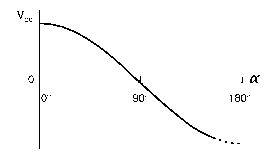Figure 4.6 Average d.c. output voltage from a fully-controlled thyristor converter as a function of the firing angle delay a be further reduced to compensate and allow full d.c. voltage to be maintained.)
When a is greater than 90°, however, the output voltage is negative, as indicated by equation (2.5), and is shown in Figure 4.6. A single fully controlled converter therefore has the potential for two-quadrant operation, though it has to be admitted that this capability is not easily exploited unless we are prepared to employ reversing switches in the armature or field circuits. This is discussed next.

### Single-converter reversing drives

We will consider a fully controlled converter supplying a permanent-magnet motor, and see how the motor can be regeneratively braked from full speed in one direction, and then accelerated up to full speed in reverse. We looked at this procedure in principle at the end of topic 3, but here we explore the practicalities of achieving it with a converter-fed drive. We should be clear from the outset that in practice, all the user has to do is to change the speed reference signal from full forward to full reverse: the control system in the drive converter takes care of matters from then on. What it does, and how, is discussed below.
When the motor is running at full speed forward, the converter delay angle will be small, and the converter output voltage V and current I will both be positive. This condition is shown in Figure 4.7(a), and corresponds to operation in quadrant 1.
In order to brake the motor, the torque has to be reversed. The only way this can be done is by reversing the direction of armature current. The converter can only supply positive current, so to reverse the motor torque we have to reverse the armature connections, using a mechanical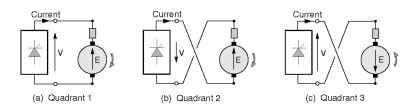Figure 4.7 Stages in motor reversal using a single-converter drive and mechanical reversing switch
switch or contactor, as shown in Figure 4.7(b). (Before operating the contactor, the armature current would be reduced to zero by lowering the converter voltage, so that the contactor is not required to interrupt current.) Note that because the motor is still rotating in the positive direction, the back e.m.f. remains in its original sense; but now the motional e.m.f. is seen to be assisting the current and so to keep the current within bounds the converter must produce a negative voltage V which is just a little less than E. This is achieved by setting the delay angle at the appropriate point between 90° and 180°. (The dotted line in Figure 4.6 indicates that the maximum acceptable negative voltage will generally be somewhat less than the maximum positive voltage: this restriction arises because of the need to preserve a margin for commutation of current between thyristors.) Note that the converter current is still positive (i.e. upwards in Figure 4.7(b)), but the converter voltage is negative, and power is thus lowing back to the mains. In this condition the system is operating in quadrant 2, and the motor is decelerating because of the negative torque. As the speed falls, E reduces, and so V must be reduced progressively to keep the current at full value. This is achieved automatically by the action of the current-control loop, which is discussed later.
The current (i.e. torque) needs to be kept negative in order to run up to speed in the reverse direction, but after the back e.m.f. changes sign (as the motor reverses), the converter voltage again becomes positive and greater than E, as shown in Figure 4.7(c). The converter is then rectifying, with power being fed into the motor, and the system is operating in quadrant 3.
Schemes using reversing contactors are not suitable where the reversing time is critical, because of the delay caused by the mechanical reversing switch, which may easily amount to 200-400 msec. Field reversal schemes operate in a similar way, but reverse the field current instead of the armature current. They are even slower, because of the relatively long time-constant of the field winding.

### Double-converter reversing drives

Where full four-quadrant operation and rapid reversal is called for, two converters connected in anti-parallel are used, as shown in Figure 4.8. One converter supplies positive current to the motor, while the other supplies negative current.
The bridges are operated so that their d.c. voltages are almost equal thereby ensuring that any d.c. circulating current is small, and a reactor is placed between the bridges to limit the low of ripple currents which result from the unequal ripple voltages of the two converters. Alternatively, the reactor can be dispensed with by only operating one converter at a time. The changeover from one converter to the other can only take place after the firing pulses have been removed from one converter, and the armature current has decayed to zero. Appropriate zero-current detection circuitry is provided as an integral part of the drive, so that as far as the user is concerned, the two converters behave as if they were a single ideal bidirectional d.c. source.
Prospective users need to be aware of the fact that a basic single converter can only provide for operation in one quadrant. If regenerative braking is required, either field or armature reversing contactors will be needed; and if rapid reversal is essential, a double converter has to be used. All these extras naturally push up the purchase price.

### Power factor and supply effects

One of the drawbacks of a converter-fed d.c. drive is that the supply power factor is very low when the motor is operating at high torque (i.e. high current) and low speed (i.e. low armature voltage), and is less than unity even at base speed and full load. This is because the supply current waveform lags the supply voltage waveform by the delay angle a,as shown (for a 3-phase converter) in Figure 4.9, and also the supply current is approximately rectangular (rather than sinusoidal).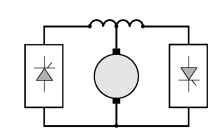Figure 4.8 Double-converter reversing drive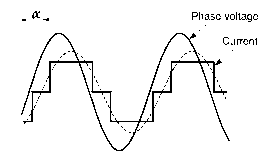Figure 4.9 Supply voltage and current waveforms for single-phase converter-fe d.c. motor drive
It is important to emphasise that the supply power factor is always lagging, even when the converter is inverting. There is no way of avoiding the low power factor, so users of large drives need to be prepared to augment their existing power factor correcting equipment if necessary.
The harmonics in the mains current waveform can give rise to a variety of interference problems, and supply authorities generally impose statutory limits. For large drives (say hundreds of kilowatts), filters may have to be provided to prevent these limits from being exceeded.
Since the supply impedance is never zero, there is also inevitably some distortion of the mains voltage waveform, as shown in Figure 4.10 which indicates the effect of a 6-pulse converter on the supply line-to-line voltage waveform. The spikes and notches arise because the mains is momentarily short-circuited each time the current commutates from one thyristor to the next, i.e. during the overlap period discussed earlier. For the majority of small and medium drives, connected to stiff industrial supplies, these notches are too small to be noticed (they are greatly exaggerated for the sake of clarity in Figure 4.10); but they can pose a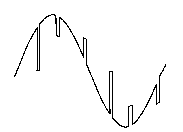Figure 4.10 Distortion of line voltage waveform caused by overlap in three-phase fully-controlled converter.(The width of the notches has been exaggerated for the sake of clarity.) serious interference problem for other consumers when a large drive is connected to a weak supply.

Previous post: